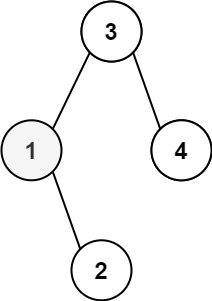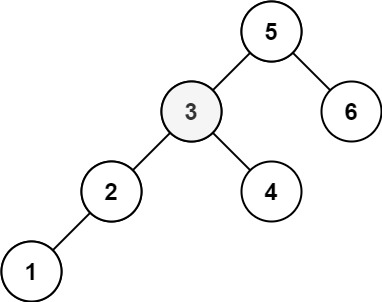# GeetCode Hub

Given the `root` of a binary search tree, and an integer `k`, return the `kth` (1-indexed) smallest element in the tree.

Example 1:```Input: root = [3,1,4,null,2], k = 1
Output: 1
```

Example 2:```Input: root = [5,3,6,2,4,null,null,1], k = 3
Output: 3
```

Constraints:

• The number of nodes in the tree is `n`.
• `1 <= k <= n <= 104`
• `0 <= Node.val <= 104`

Follow up: If the BST is modified often (i.e., we can do insert and delete operations) and you need to find the kth smallest frequently, how would you optimize?

/** * Definition for a binary tree node. * public class TreeNode { * int val; * TreeNode left; * TreeNode right; * TreeNode() {} * TreeNode(int val) { this.val = val; } * TreeNode(int val, TreeNode left, TreeNode right) { * this.val = val; * this.left = left; * this.right = right; * } * } */ class Solution { public int kthSmallest(TreeNode root, int k) { } }